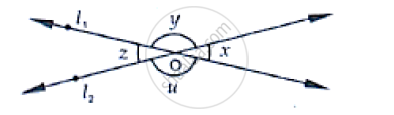# In the Below Fig, Lines �1 and �2 Intersect at O, Forming Angles as Shown in the Figure. If X = 45, Find the Values of X, Y, Z and U. - Mathematics

In the below fig, lines �1 and �2 intersect at O, forming angles as shown in the figure. If x = 45, Find the values of x, y, z and u.#### Solution

Given that

x = 45°, y = ?, z = ?, u = ?

Vertically opposite sides are equal

∴z = x = 45°

z and u angles are linear pair of angles

z + u = 180°

z = 180° - 4

⇒ u = 180° - x

⇒u = 180° - 45°                   [∵  x = 45°]

⇒ u = 135°

x and y angles are linear pair of angles

∴x + y = 180°

y = 180° - x

y = 180° - 45°

y = 135°

∴x = 45°, y = 135°, z = 135° and u = 45°

Concept: Concept of Parallel Lines
Is there an error in this question or solution?

#### APPEARS IN

RD Sharma Mathematics for Class 9
Chapter 10 Lines and Angles
Exercise 10.3 | Q 1 | Page 22